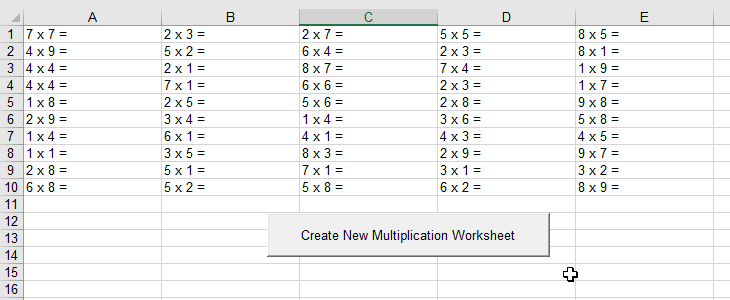top of page
Search

# Create A Multiplication Table Worksheet in Excel

Updated: Nov 9, 2019

Are you a primary school Math teacher who is teaching multiplication table? Are you parents who are gonna give your kids some multiplication exercises? This section is right here for you. We are gonna help you create multiplication table worksheets from Excel."Mathematics is the key and door to the sciences." - Galileo Galilei

When the kids start to learn multiplication table, they might need some exercises to master it. To manually write the incomplete number sentences like 9x9=, 8x8=, 7x7= etc. will be troublesome. Let's try to use Excel to give you a more efficient way to create those automatically. That really saves a lot of time.

In the following example, we will use the Rnd function. It returns a value less than 1 but greater than or equal to zero. The returned value is a pseudorandom number. Let's say we want to create a single digit multiplication table worksheet. The lowest number is 1 while the highest number is 9. We may use the below formula to produce the random integers between 1 to 9.

Int((UpperBound - LowerBound + 1) * Rnd + LowerBound)

Here, UpperBound is 9 while LowerBound is 1.

Simply add a command button on your Excel sheet then rename it as "Create New Multiplication Worksheet". Then copy the below codes to your VBA code pane under the corresponding sheet.

Private Sub CommandButton1_Click()

Dim i, j, UpperBound, LowerBound As Integer

UpperBound = 9

LowerBound = 1

For i = 1 To 10

For j = 1 To 5

ActiveSheet.Cells(i, j) = Int((UpperBound - LowerBound + 1) * Rnd + LowerBound) & " x " & Int((UpperBound - LowerBound + 1) * Rnd + LowerBound) & " ="

Next j

Next i

End Sub

Well, let's click the button and see how it works.